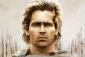# Physics

3 posts / 0 new
Theresa Lopez M...Physics

A mass of 20kg is projected up an inclined of 26 degrees with a velocity of 4 m/s. If the coefficient of friction is 0.2, find i) the maximum distance that the package will move along the plane. and ii) what will be the velocity of the package when it comes back to initial position.

AlexanderEnergy equation for going upward
E1 - Elost = E2
1/2 mv12 - Friction x s = mgh
1/2 (20)(42) - 0.2(20 cos 26deg)(9.81) s = 20(9.81)(s sin 26deg)
s = 1.32 m

For going downward, v1 = 4 m/sec

Theresa Lopez M...Thank you po.

• Mathematics inside the configured delimiters is rendered by MathJax. The default math delimiters are $$...$$ and $...$ for displayed mathematics, and $...$ and $...$ for in-line mathematics.